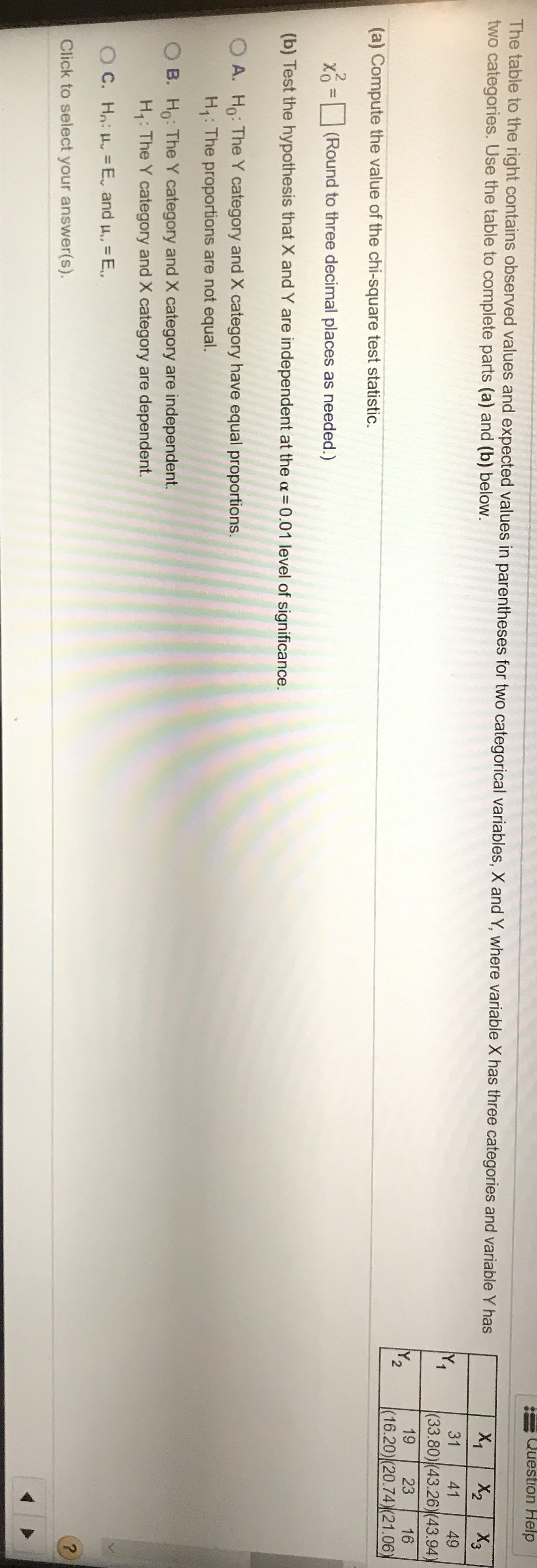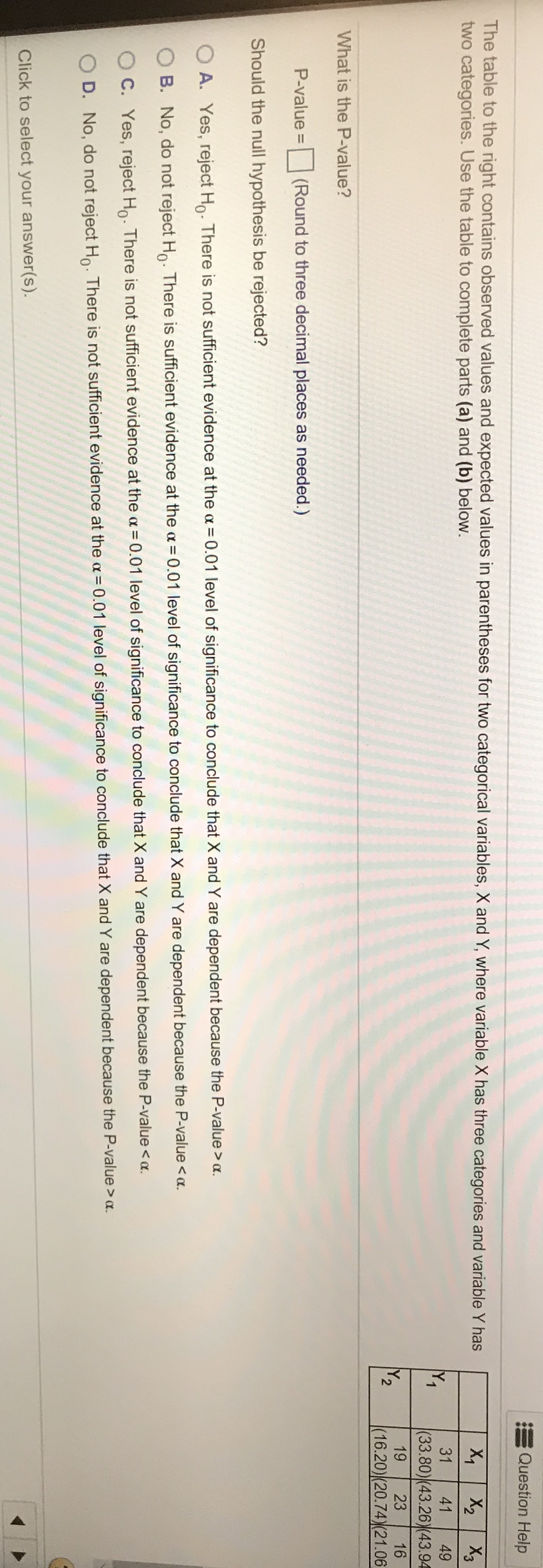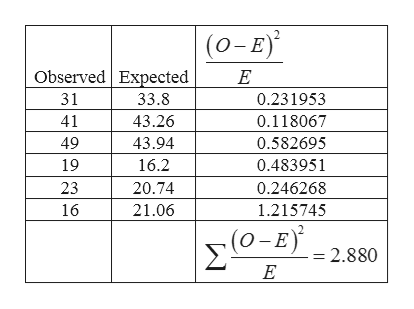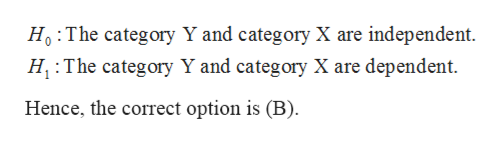# Question HelpThe table to the right contains observed values and expected values in parentheses for two categorical variables, X and Y, where variable X has three categories and variable Y hastwo categories. Use the table to complete parts (a) and (b) below.X2 X3X1314149Y1|(33.80)(43.26(43.94)192316Y2|(16.20)(20.74) (21.06)(a) Compute the value of the chi-square test statistic.x -(Round to three decimal places as needed.)(b) Test the hypothesis that X and Y are independent at the a =0.01 level of significance.OA. Ho: The Y category and X category have equal proportions.H: The proportions are not equal.B. Ho: The Y category and X category are independent.Hi: The Y category and X category are dependent.OC.H= E, and u, EClick to select your answer(s). Question HelpThe table to the right contains observed values and expected values in parentheses for two categorical variables, X and Y, where variable X has three categories and variable Y hastwo categories. Use the table to complete parts (a) and (b) below.X X2 X3314149Y1|(33.80)(43.26)(43.94192316Y2(16.20) (20.74)(21.06What is the P-value?P-value =(Round to three decimal places as needed.)Should the null hypothesis be rejected?O A. Yes, reject Ho. There is not sufficient evidence at the a0.01 level of significance to conclude that X and Y are dependent because the P-value>aB. No, do not reject Ho. There is sufficient evidence at the a = 0.01 level of significance to conclude that X and Y are dependent because the P-value < .C. Yes, reject Ho There is not sufficient evidence at the a 0.01 level of significance to conclude that X and Y are dependent because the P-value < .O D. No, do not reject HThere is not sufficient evidence at the a 0.01 level of significance to conclude that X and Y are dependent because the P-value > a.Click to select your answer(s).

Question
64 views

Helphelp_outlineImage TranscriptioncloseQuestion Help The table to the right contains observed values and expected values in parentheses for two categorical variables, X and Y, where variable X has three categories and variable Y has two categories. Use the table to complete parts (a) and (b) below. X2 X3 X1 31 41 49 Y1 |(33.80)(43.26(43.94) 19 23 16 Y2 |(16.20)(20.74) (21.06) (a) Compute the value of the chi-square test statistic. x - (Round to three decimal places as needed.) (b) Test the hypothesis that X and Y are independent at the a =0.01 level of significance. OA. Ho: The Y category and X category have equal proportions. H: The proportions are not equal. B. Ho: The Y category and X category are independent. Hi: The Y category and X category are dependent. OC.H = E, and u, E Click to select your answer(s). fullscreenhelp_outlineImage TranscriptioncloseQuestion Help The table to the right contains observed values and expected values in parentheses for two categorical variables, X and Y, where variable X has three categories and variable Y has two categories. Use the table to complete parts (a) and (b) below. X X2 X3 31 41 49 Y1 |(33.80)(43.26)(43.94 19 23 16 Y2 (16.20) (20.74)(21.06 What is the P-value? P-value = (Round to three decimal places as needed.) Should the null hypothesis be rejected? O A. Yes, reject Ho. There is not sufficient evidence at the a 0.01 level of significance to conclude that X and Y are dependent because the P-value>a B. No, do not reject Ho. There is sufficient evidence at the a = 0.01 level of significance to conclude that X and Y are dependent because the P-value < . C. Yes, reject Ho There is not sufficient evidence at the a 0.01 level of significance to conclude that X and Y are dependent because the P-value < . O D. No, do not reject H There is not sufficient evidence at the a 0.01 level of significance to conclude that X and Y are dependent because the P-value > a. Click to select your answer(s). fullscreen
check_circle

Step 1

The provided data can be tabulated as:help_outlineImage Transcriptionclose(о-E) Observed Expected Е 31 33.8 0.231953 41 43.26 0.118067 49 43.94 0.582695 19 16.2 0.483951 23 20.74 0.246268 1.215745 16 21.06 2.880 E fullscreen
Step 2

(a)

The value of the chi- square statistic is:

Step 3

(b)

The null and alterative...help_outlineImage TranscriptioncloseHo The category Y and category X are independent H The category Y and category X are dependent. Hence, the correct option is (B) fullscreen

### Want to see the full answer?

See Solution

#### Want to see this answer and more?

Solutions are written by subject experts who are available 24/7. Questions are typically answered within 1 hour.*

See Solution
*Response times may vary by subject and question.
Tagged in

### Statistics